• 一元二次方程复数根求解
千次阅读
2020-12-03 18:18:45

/*无聊时候写的，我想每个入门的编程都会拿着个练手把，多的不说，我们来谈谈正经的——此功能实现一元二次方程求解，复数情况，输入字符处理判断是否为数字*/

import os

import math

import cmath

/*自定义mx函数，求解，*/

def mx(a,b,c):

mm = (b ** 2) - (4 * a * c)

if mm > 0:

print('此函数有两个解')

x1=(-b+math.sqrt(mm))/(2*a)

x2 = (-b - math.sqrt(mm)) / (2 * a)

print("{:.0f}x**2+{:.0f}x+{:.0f}的结果为：x={:.0f} 和 x={:.0f}".format(a,b,c,x1,x2))

elif mm ==0:

print('此方程只有一个解')

print("{:.0f}x**2+{:.0f}x+{:.0f}的结果为：x={:.0f}".format(a, b, c, (-b+math.sqrt(mm))/(2*a)))

elif mm <0:

x1=(-b+cmath.sqrt(mm))/(2*a)

x2 = (-b - cmath.sqrt(mm)) / (2 * a)

print("{:.0f}x**2+{:.0f}x+{:.0f}的结果为：x={:.0f} 和 x={:.0f}".format(a, b, c, x1, x2))

else :

print('无解')

/*自定义is_number函数，判断用户输入的字符类型是否为数字，是为true不是为false。*/

def is_number(s):

try:

float(s)

return True

except ValueError:

pass

try:

import unicodedata

unicodedata.numeric(s)

return True

except (TypeError, ValueError):

pass

return False

/*自定义panduan函数，如果用户输入的字符类型为数字，返回数字，如果不是数字，则提示要求重新输入知道输入的为字符为止，(提示这里用while来实现循环，如果用户输入的一直不是数字，将会一直走不出while,最好用or一下用户输入的最大次数，即是用户输入的最大次数)。*/

def panduan(xx):

if is_number(xx)==True:

return xx

else:

while is_number(xx)==False:

print('不是数字，请再次输入')

cc =input("请输入一个数:")

if is_number(cc)==True:

break

return cc

/*主程序——input输入数字，先用is_number判断是否为数字，如果不是，返回false，再用panduan来再次依靠is_number的值，来进行判断为false则要求用户重新输入，为true则返回用户输入的数字，其中在python中input的数字为字符型，需要用float来转换成数字。 */

print('此程序用于计算一元二次方程解,请依次输入三个数')

zz=input("是否要开始计算:yes/任意退出:")

while zz =='yes':

a=float(panduan(input("请输入第一个数:")))

b=float(panduan(input("请输入第二个数:")))

c=float(panduan(input("请输入第三个数:")))

mx(a,b,c)

zz = input("是否还要继续计算:yes/no——:")

if zz!='yes':

qq =input('确认退出计算界面？yes/任意键继续')

if qq == 'yes':

print('感谢使用。' ,end="")

else:

zz=input("是否要开始计算:yes/任意退出:")

else:

zz = input("是否继续计算:yes/no——:")

更多相关内容
• 可以计算一元二次方程复数根的模拟计算机 模拟计算机采用模拟电路计算加减乘除。例如计算，（28+80-65）×56÷79，可以先用直流电源电路产生一个DC28V,1A的信号，这个信号代表实数28。再用直流电源电路产生一个DC80V...

可以计算一元二次方程复数根的模拟计算机

模拟计算机采用模拟电路计算加减乘除。例如计算，（28+80-65）×56÷79，可以先用直流电源电路产生一个DC28V,1A的信号，这个信号代表实数28。再用直流电源电路产生一个DC80V,1A的信号，这个信号代表实数80。再用直流电源电路产生一个DC65V,1A的信号，这个信号代表实数65。再用直流电源电路产生一个DC56V,1A的信号，这个信号代表实数56。再用直流电源电路产生一个DC79V,1A的信号，这个信号代表实数79。注意这几个直流稳压电路都是由硅堆将AC220V电源变为直流电源，电源由调压器调节，电流固定不变一直为1A。AC220V是由AC380V经过变压器和水银引燃管变压得到。这样做的好处是，电压电流的大小可以由水银引燃管调节。电路下载网址：链接: https://pan.baidu.com/s/1JbWESaLKwpWnMWbZGdNoLQ 提取码: ufy8

https://share.weiyun.com/eXVCG2E3

这个电源电路如下：

本地图片，请重新上传

同时，AC220V电源经过变压器变压，调压器调压，可以将电压升高到AC10000V，这样可以产生DC10000V,1A的信号，也就是说这个电源可以产生1到10000的实数。把调压器的中点接地，比地线高的电位，带正电，表示正数，比地线低的电位，带负电，表示负数。这个可以产生负电位，正电位的调压器电路如下图所示。电路的末端接上电流表电压表，可以测量电路输出的电压，电流，让电流一直保持1A，电压是线性的直流电。

本地图片，请重新上传

由调压器电路得到这些电压数字后，再把它们接到加法器电路，减法器电路，乘法器电路，除法器电路，就会得到上面运算式（28+80-65）×56÷79的计算结果26.9367。

加法器电路如下

本地图片，请重新上传

加法器电路如下

本地图片，请重新上传

乘法器电路如下

本地图片，请重新上传

本地图片，请重新上传

本地图片，请重新上传

除法器电路如下

本地图片，请重新上传

集成运放电路如下

本地图片，请重新上传

本地图片，请重新上传

本地图片，请重新上传

本地图片，请重新上传

本地图片，请重新上传

本地图片，请重新上传

本地图片，请重新上传

本地图片，请重新上传

本地图片，请重新上传

本地图片，请重新上传

上面整个过程的计算电路如下

本地图片，请重新上传

一元二次方程ax²+bx+c=0（a≠0）,它的求根公式是x=[-b±√(b²-4ac)]/2a。

例如计算下面的一元二次方程x²+3x+2=0，根据求根公式，它的根是2,1。

利用上面的调压器电路产生一个不固定可变电压，电流保持1A的信号，用这个信号表示方程式的自变量X，再用上面的调压器电路产生一个DC3V,1A的信号，这个信号表示常数3，再用上面的调压器电路产生一个DC3V,1A的信号，这个信号表示常数2，最后利用乘方电路，乘法器电路，加法器电路将上面的几个电压连接到一起，最后的输出端接上电压表，电流表。调节电源电路里面的电位器，使表示X的电源输出得电压呈线性变化，电流保持不变为DC1A，最后电路输出端的电压为DC0V，1A时，这时表示X的电源输出的电压值为DC2V或者DC1V，表示方程的一个根是2，1。上面计算一元二次方程的电路如下。

本地图片，请重新上传

同时计算二元二次方程组， 3x²-y² =8

                    ｛ x-y =0


得X1=2,Y1=-2,X2=-2,Y2=2。可以将两个方程相加得

3x²-y² +x-y=8,方程式两边同减8得

3x²-y² +x-y-8=0。这个方程就是我们需要用模拟电路表示的方程。当这个电路的输出为0V，1A时，X，Y的电压值就是方程的根。

利用上面的调压器电路产生一个不固定可变电压，电流保持1A的信号，用这个信号表示方程式的自变量X，利用上面的调压器电路产生另外一个不固定可变电压，电流保持1A的信号，用这个信号表示方程式的自变量Y，再用上面的调压器电路产生一个DC8V,1A的信号，这个信号表示常数8，最后利用乘方电路，加法器电路，减法器电路将上面的几个电压连接到一起，乘法电路就是一个数乘上同一个数的乘法电路。在最后的输出端接上电压表，电流表。调节电源电路里面的电位器，使表示X的电源输出得电压呈线性变化，电流保持不变为DC1A，使表示X的电源输出得电压呈线性变化，调节电源电路里面的电位器，使表示Y的电源输出得电压呈线性变化，电流保持不变为DC1A，使表示Y的电源输出得电压呈线性变化，电流保持不变为DC1A，最后的输出端的电压为DC0V，1A时，这时表示X的电源输出的电压值为DC2V或者DC-2V，表示X的电源输出的电压值为DC-2V或者DC2V，表示方程的根是X1=2,Y1=-2,X2=-2,Y2=2。上面计算一元二次方程的电路如下。

本地图片，请重新上传

一元二次方程ax²+bx+c=0（a≠0）,它的求根公式是x=[-b±√(b²-4ac)]/2a。当b²-4ac＜0时有两个虚数根，当b²-4ac=0时，有一个实数根，当b²-4ac＞0时，有两个实数根，计算x²-5x+2=0，它有两个虚数根-2.5+√17i/2,-2.5-√17i/2,复数用模拟电路表示时，电压代表复数的实部，电流代表复数的虚部，电压DC0V代表实数0，直流负电压代表复数，直流正电压代表正数。电流1A代表虚数0，直流小于1A的电流代表负虚数，直流大于1A的电流代表正虚数。还是上面的稳压电源，再加上调压电路，调节电位器，使电源输出的电压电流是直流可调，并呈线性变化。这个电源输出端接有电压表，电流表，它们可以显示电源输出的信号，这样这个电源就可以表示复数了。复数的乘法器和实数的乘法器不同，实数的乘法器只是电压的相乘，复数的乘法器是电压和电流相乘的组合。

由于c是电压信号，利用电压变电流电路，将c变为电流信号，两个信号呈线性关系。再采用电流乘法器，得到bc的电流乘积。

上面的描述可以表示为下面的框图。

本地图片，请重新上传

复数的乘法电路如下。

本地图片，请重新上传

电压变电流电路

本地图片，请重新上传

本地图片，请重新上传

电流乘法器

本地图片，请重新上传

本地图片，请重新上传

本地图片，请重新上传

电流减法器

本地图片，请重新上传

电流加法器

本地图片，请重新上传

电流变电压电路

本地图片，请重新上传

电流电压调节电路

本地图片，请重新上传

上面求虚数根的一元二次方程模拟计算机的电路图如下所示。

本地图片，请重新上传

可以看做第一个数的实部a和第二个数的实部c的乘积ac,再加上第一个数的虚部b和第二个数的虚部d的乘积bd,由于bd是电流乘积，所以要采用电流乘法器，再利用电流变电压电路，将这个电流信号变为电压信号，两个信号呈线性关系。在利用电压加法器就可以得到ac+bd的电压信号，

可以看做第二个数的实部c和第二个数的实部c的乘积cc,再加上第二个数的虚部d和第二个数的虚部d的乘积dd,由于dd是电流乘积，所以要采用电流乘法器，再利用电流变电压电路，将这个电流信号变为电压信号，两个信号呈线性关系。在利用电压加器就可以得到cc+d*d的电压信号。

再利用电压除法器，将上面两个电压相处，得到两个复数相处的结果的实部(ac+bd)/(cc+dd)。

可以看做第一个数的虚部b和第二个数的实部c的乘积bc, 由于c是电压信号，利用电压变电流电路，将这个电压信号变为电流信号，两个信号呈线性关系。再利用电流乘法器得到乘积bci。

可以看做第二个数的实部c和第二个数的实部c的乘积cc,再加上第二个数的虚部d和第二个数的虚部d的乘积dd,由于cc是电流乘积，所以要采用电压乘法器，再利用电压变电流电路，将这个电压信号变为电流信号，两个信号呈线性关系。在利用电流加器就可以得到cc+d*d的电流信号。

再利用电流除法器，将上面两个电流相处，得到两个复数相处的结果的虚部(ac+bd)/(cc+dd)。

再加上第一个数的虚部b和第二个数的虚部d的乘积bd,由于bd是电流乘积，所以要采用电流乘法器，再利用电流变电压电路，将这个电流信号变为电压信号，两个信号呈线性关系。在利用电压加法器就可以得到ac+bd的电压信号，

可以看做第二个数的实部c和第二个数的实部c的乘积cc,再加上第二个数的虚部d和第二个数的虚部d的乘积dd,由于dd是电流乘积，所以要采用电流乘法器，再利用电流变电压电路，将这个电流信号变为电压信号，两个信号呈线性关系。在利用电压加器就可以得到cc+d*d的电压信号。

再利用电压除法器，将上面两个电压相处，得到两个复数相处的结果的实部(ac+bd)/(cc+dd)。

上面的描述可以表示为下面的框图。

本地图片，请重新上传

电流除法器

本地图片，请重新上传

电压除法器

本地图片，请重新上传

本地图片，请重新上传

本地图片，请重新上传

本地图片，请重新上传

电压乘法器

本地图片，请重新上传

计算带根号一元二次方程√(x²-5)- √(x²-8)-1=0,得根为2，-2.。利用上面的调压器电路产生一个不固定可变电压，电流保持1A的信号，用这个信号表示方程式的自变量X，再用上面的调压器电路产生一个DC5V,1A的信号，这个信号表示常数5，再用上面的调压器电路产生一个DC8V,1A的信号，这个信号表示常数8，最后利用乘方电路，开方电路，减法器电路将上面的几个电压连接到一起，最后的输出端接上电压表，电流表。调节电源电路里面的电位器，使表示X的电源输出得电压呈线性变化，电流保持不变为DC1A，最后电路输出端的电压为DC0V，1A时，这时表示X的电源输出的电压值为DC2V或者DC-2V，表示方程的一个根是2，-2。上面计算一元二次方程的电路如下。

本地图片，请重新上传

电压开方电路

本地图片，请重新上传

本地图片，请重新上传

本地图片，请重新上传

本地图片，请重新上传

本地图片，请重新上传

电压减法器

本地图片，请重新上传

电压加法器

本地图片，请重新上传

图片

图片

图片

图片

图片

图片

图片

图片

图片

图片

图片

图片

图片

图片

图片

图片

图片

图片

图片

图片

图片

图片

图片

图片

图片

图片

图片

图片

图片

图片

图片

图片

图片

图片

图片

图片

图片

图片

图片

图片

图片

图片

图片

图片

图片

图片

图片

图片

图片

图片

图片

图片

图片

图片

图片

图片

图片

图片

图片

图片

图片

图片

图片

图片

图片

图片

图片

图片

图片

图片

图片

图片

图片

图片

图片

图片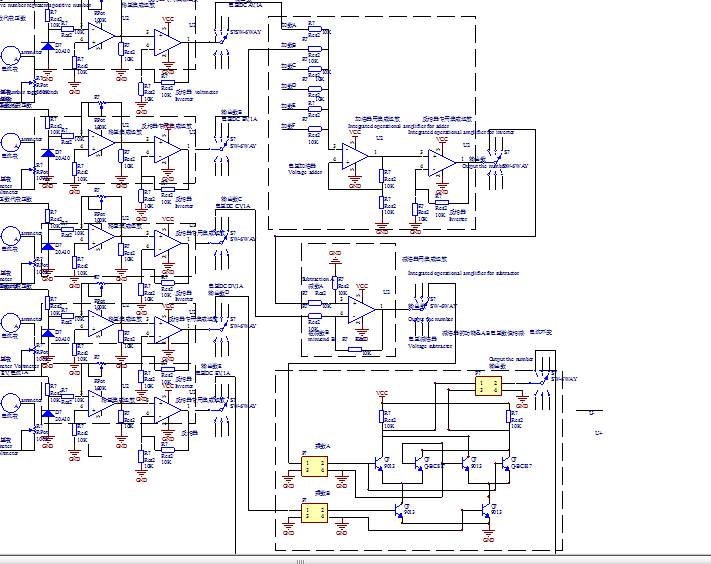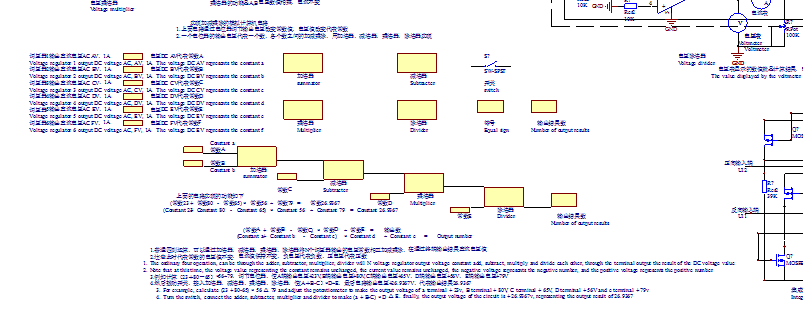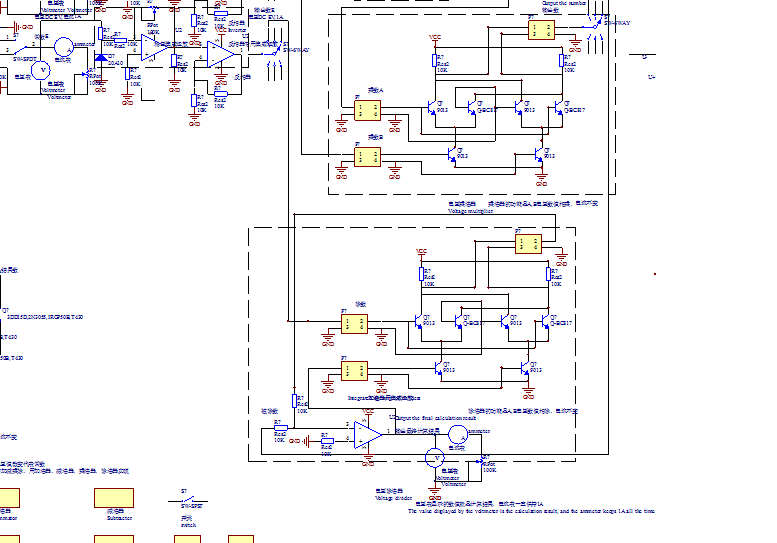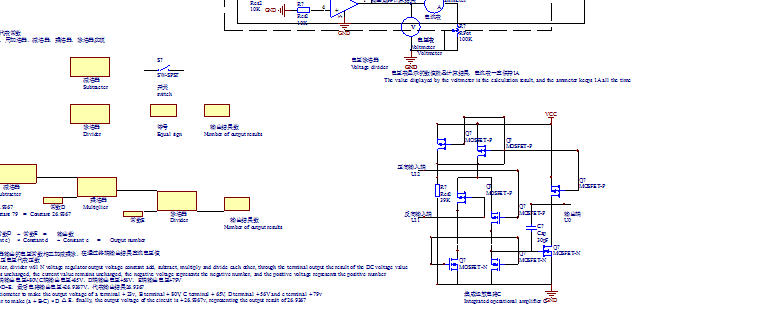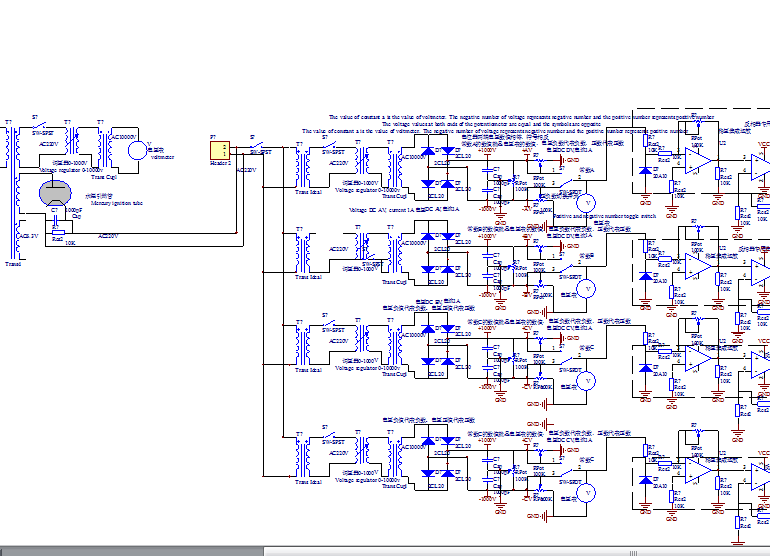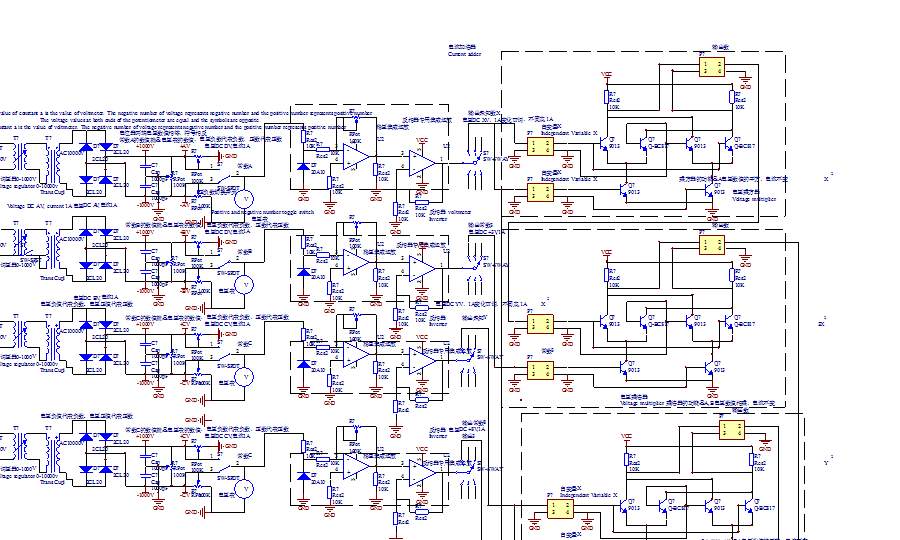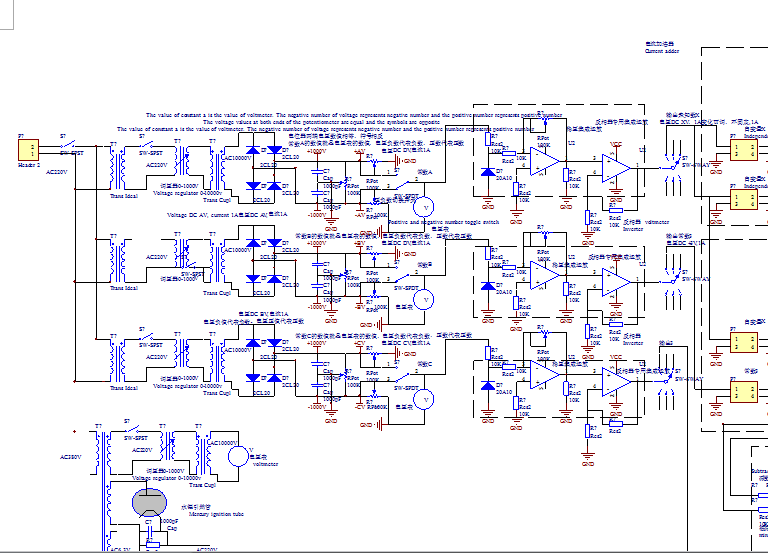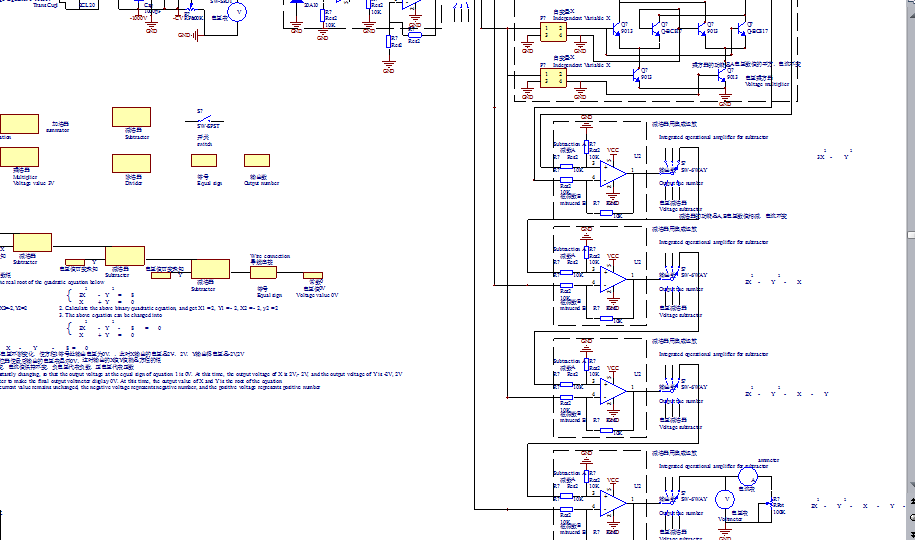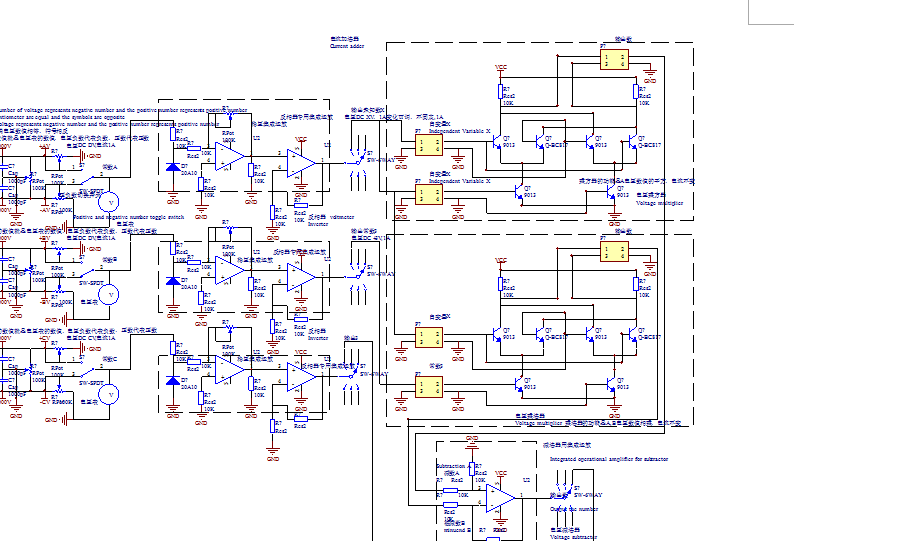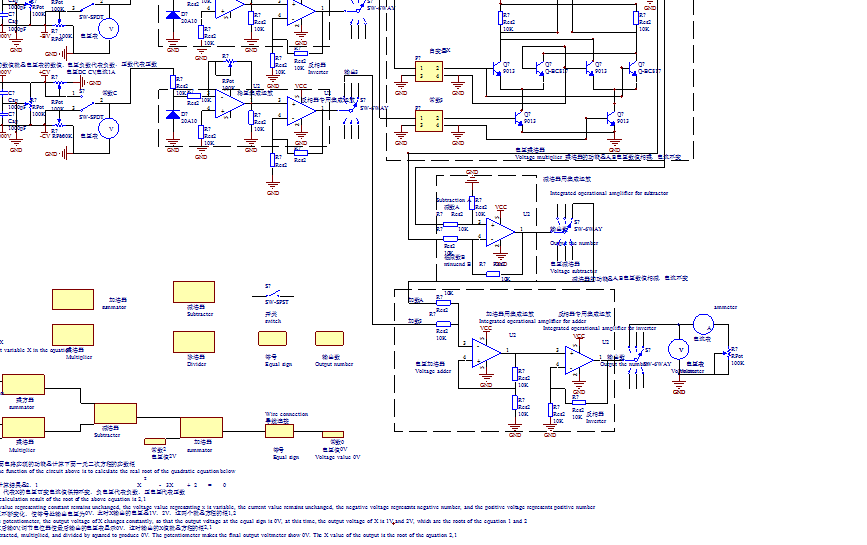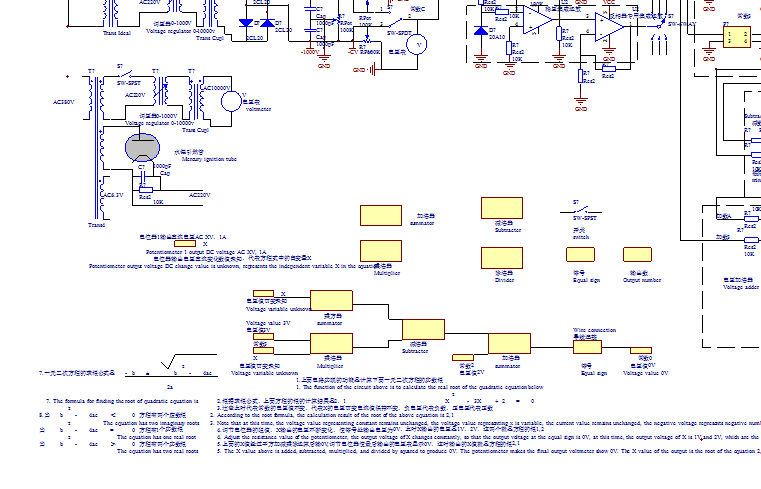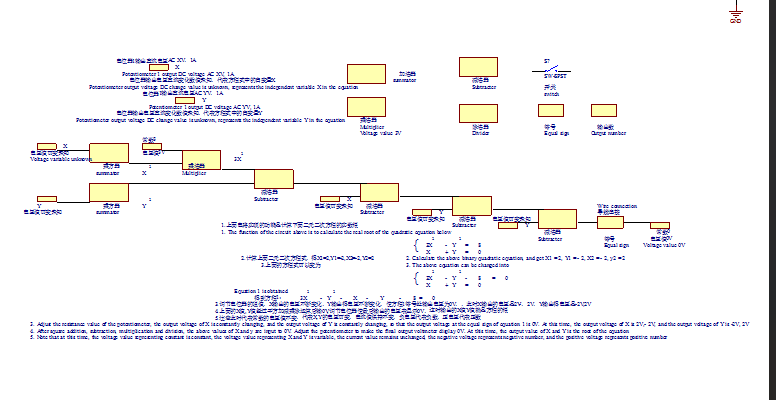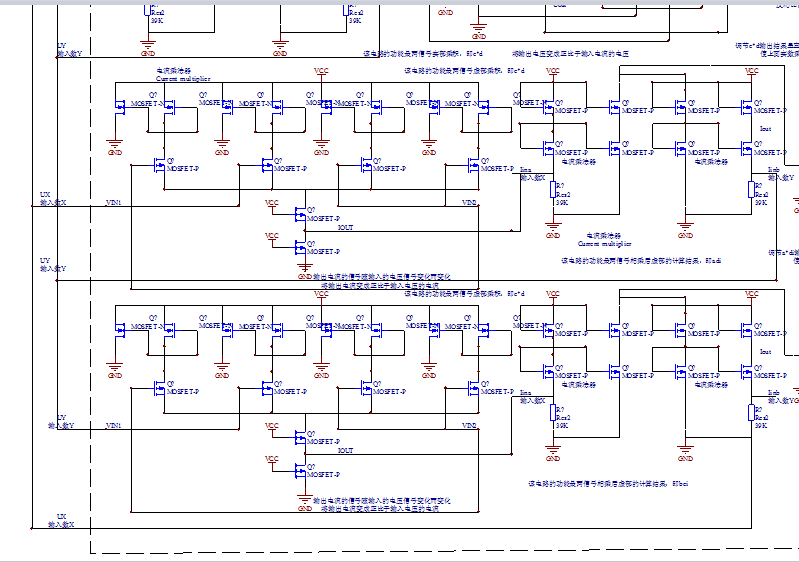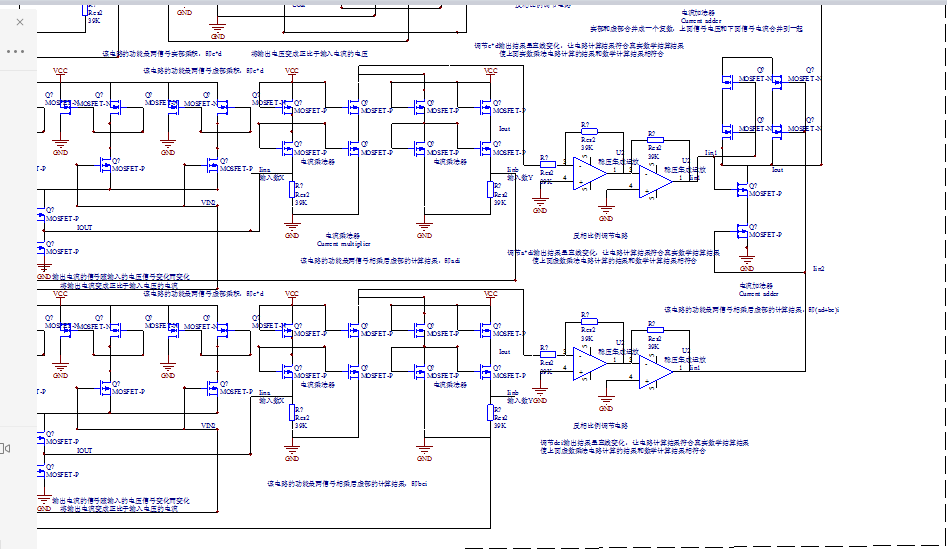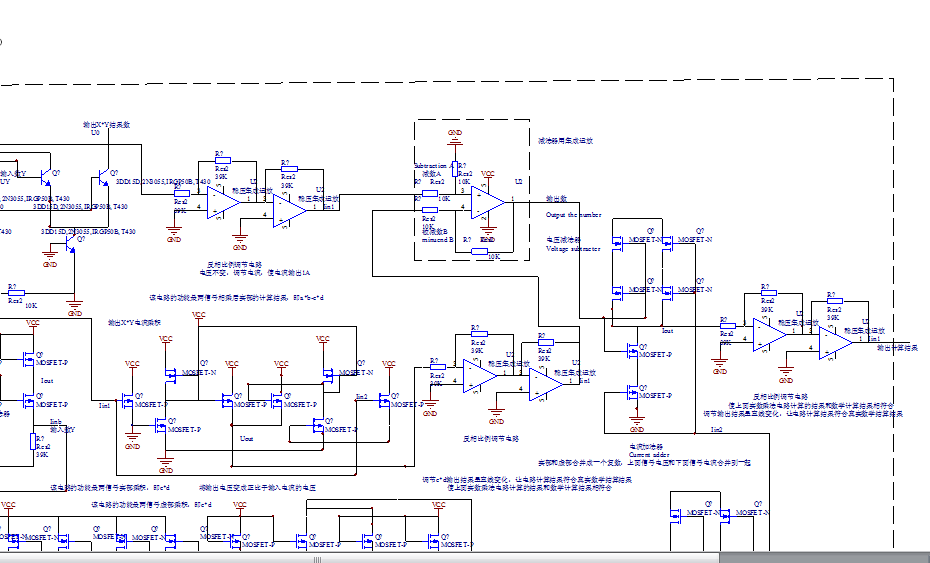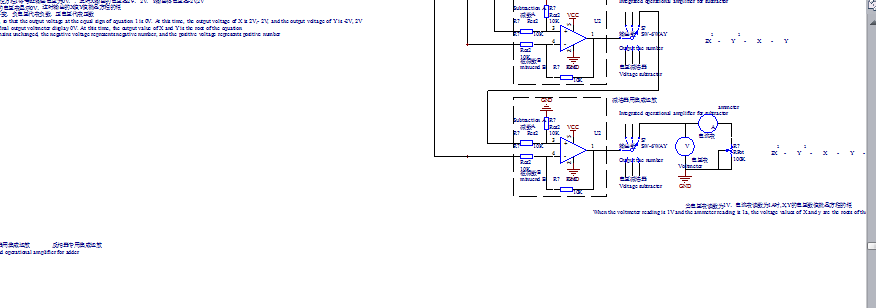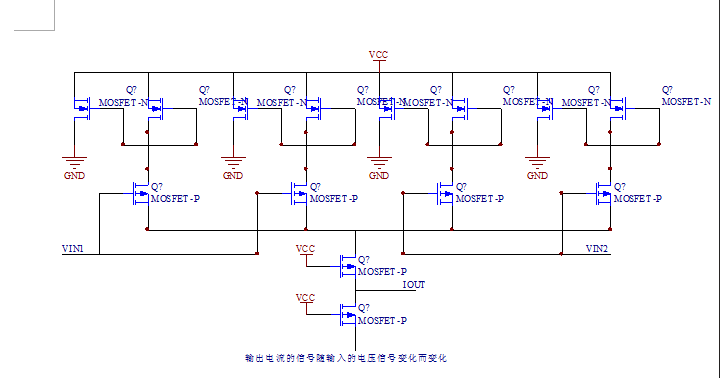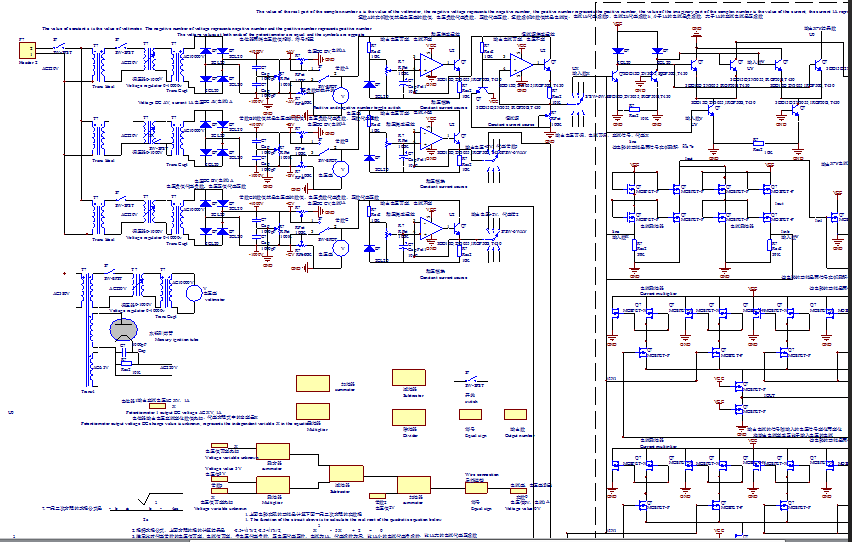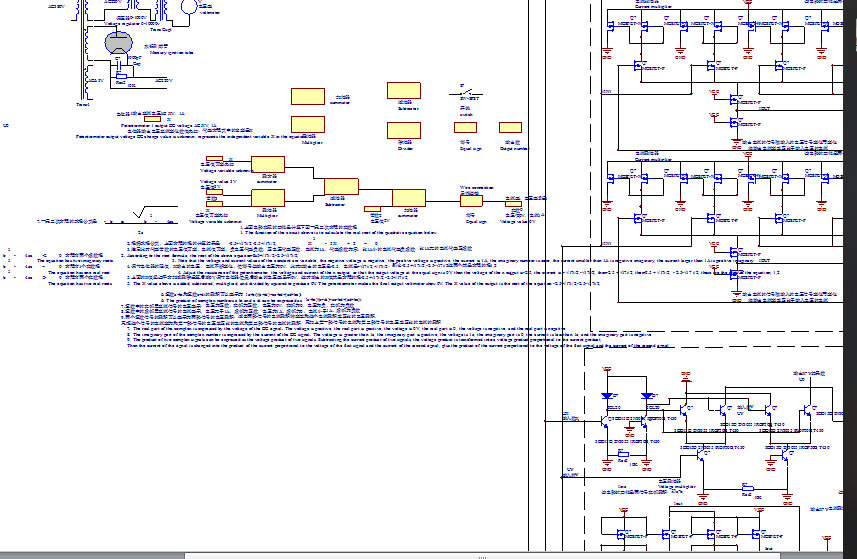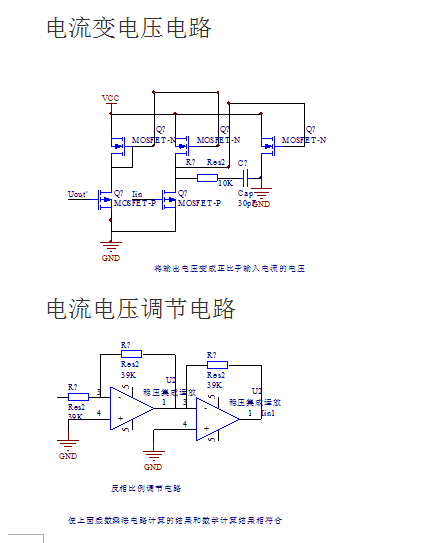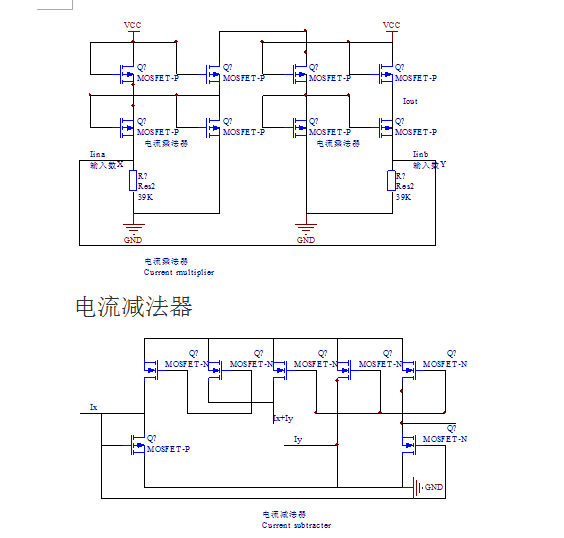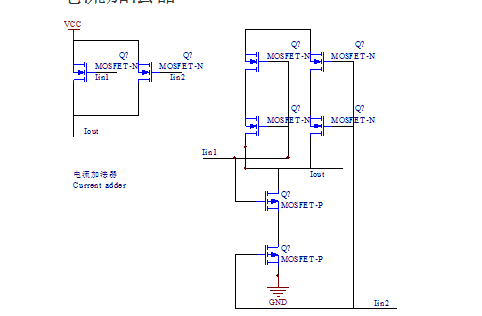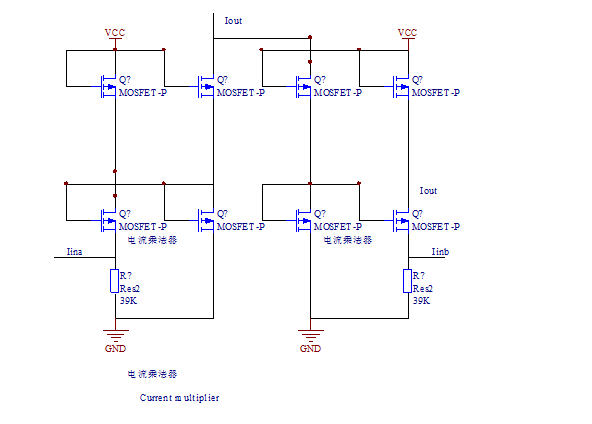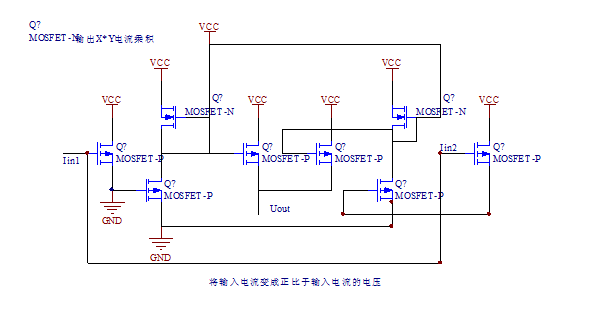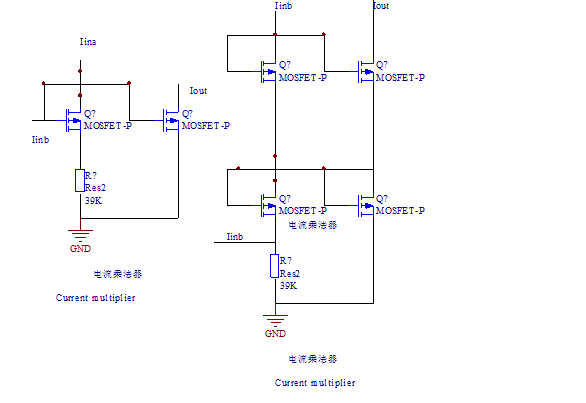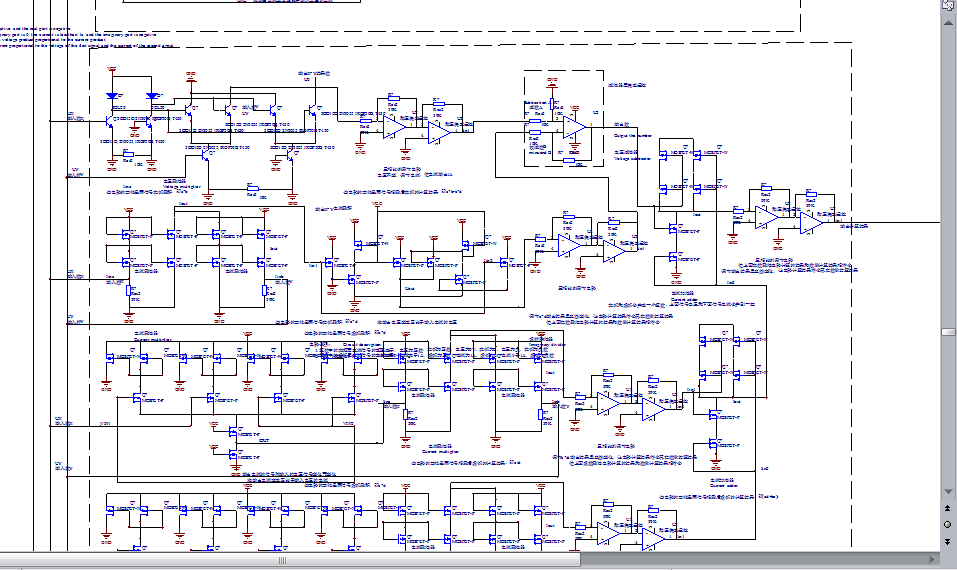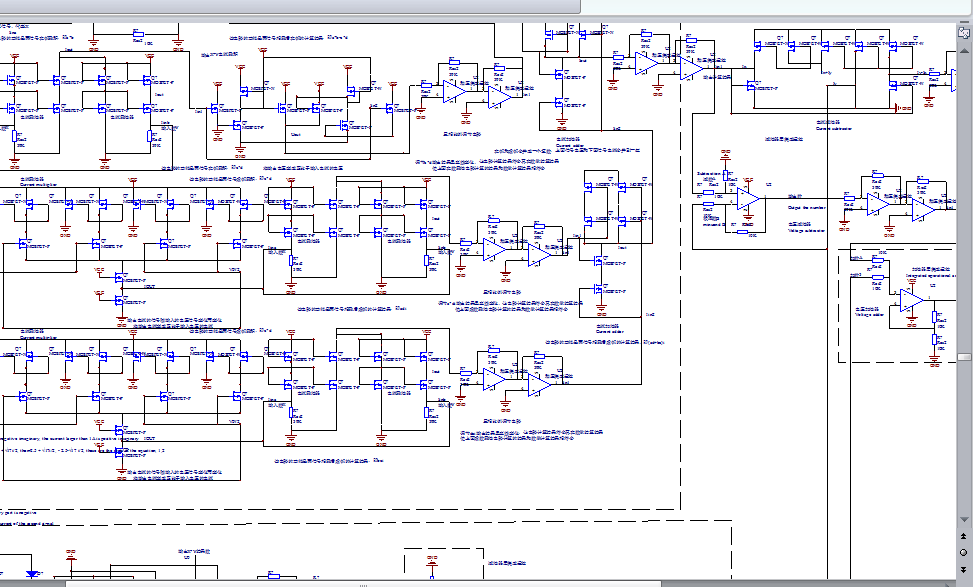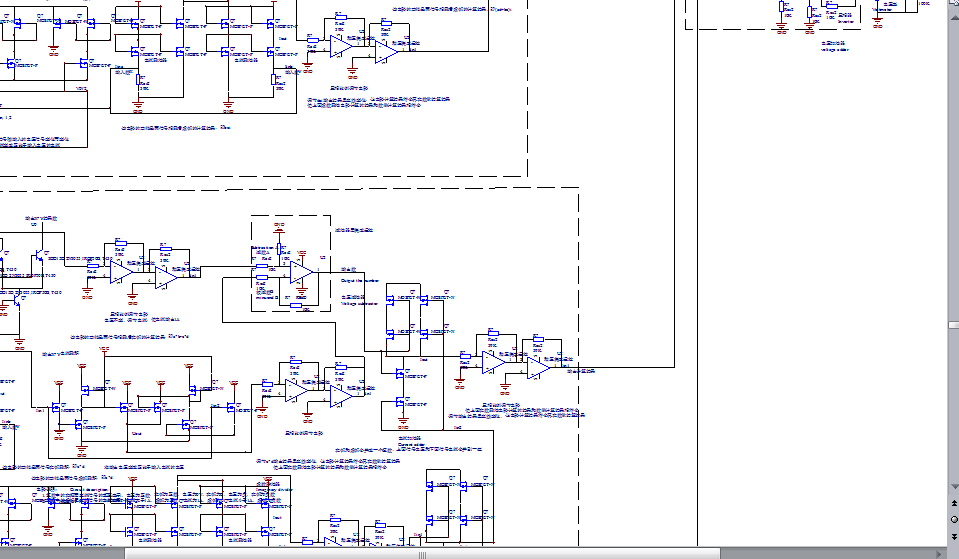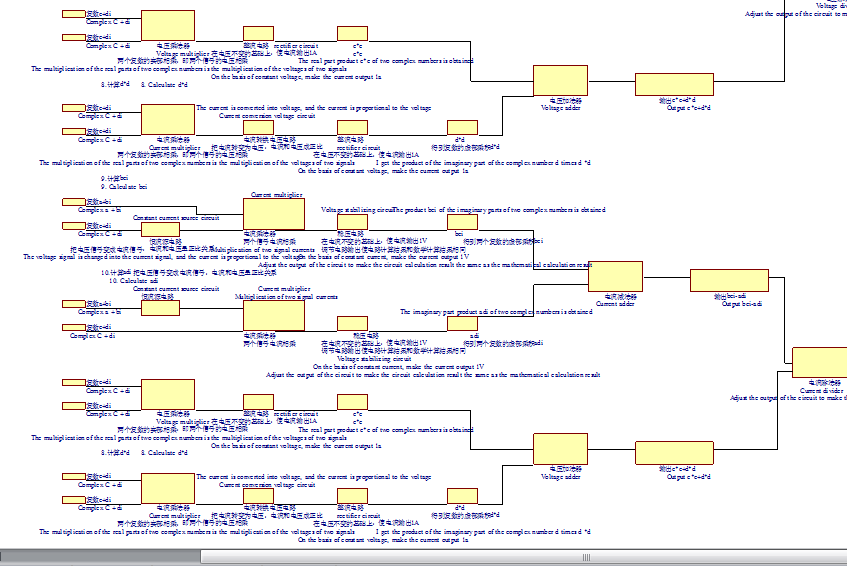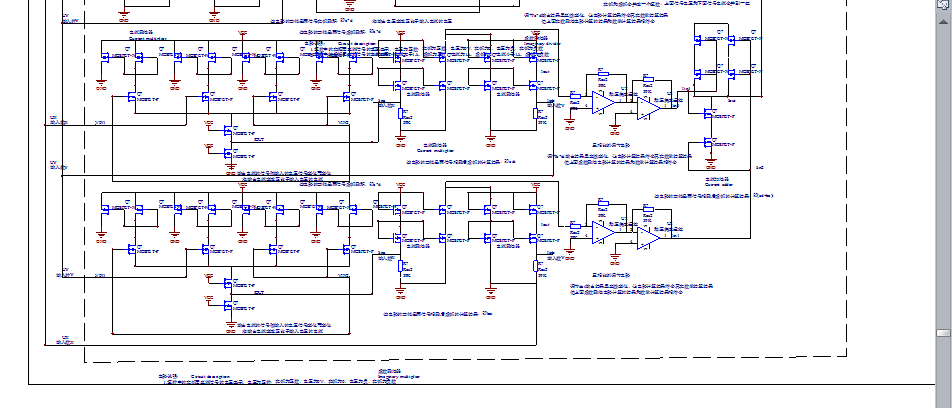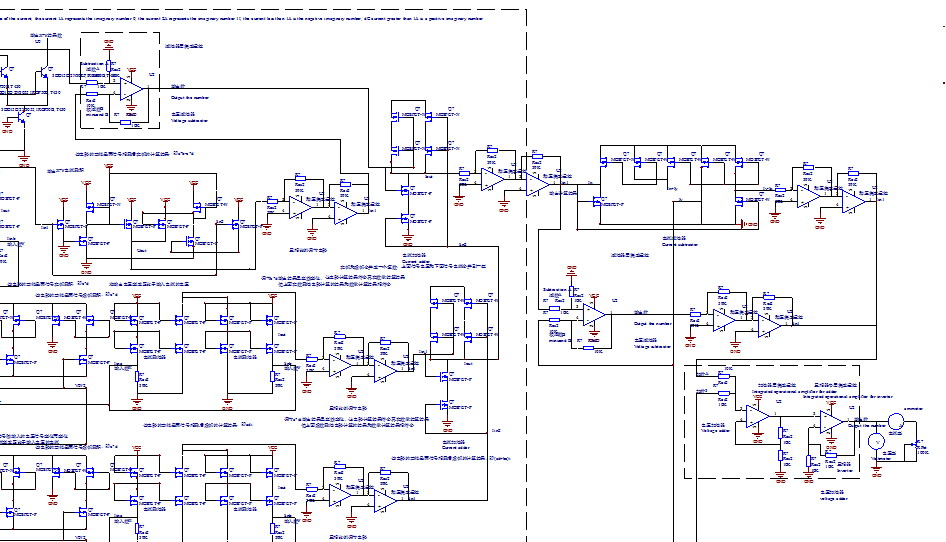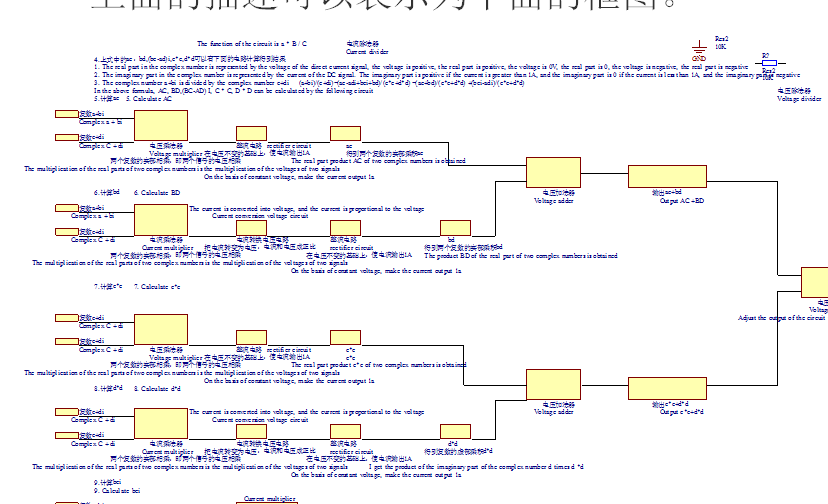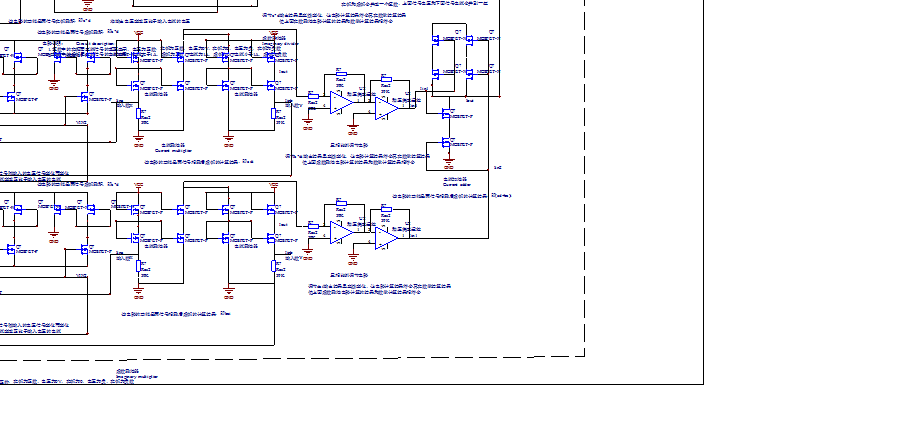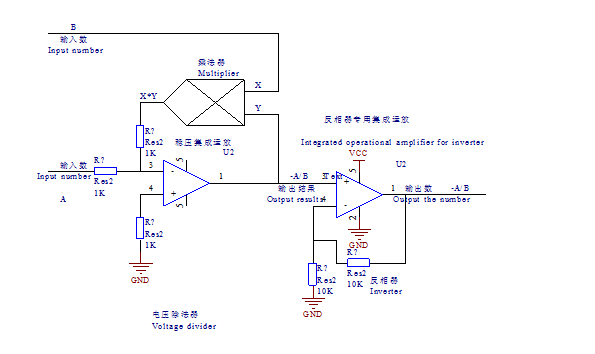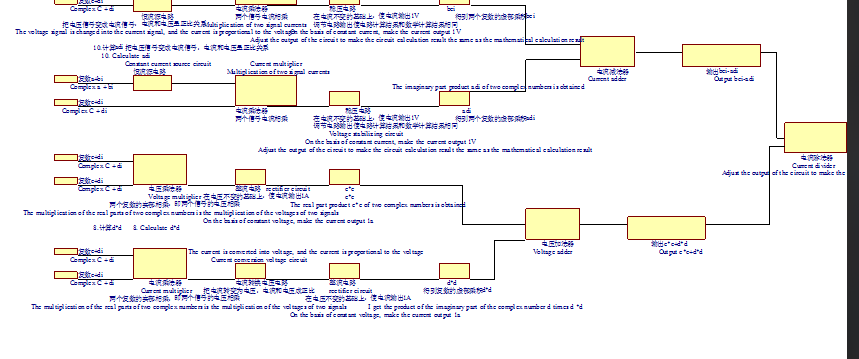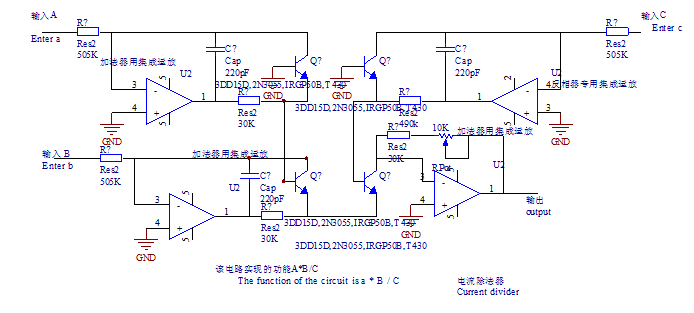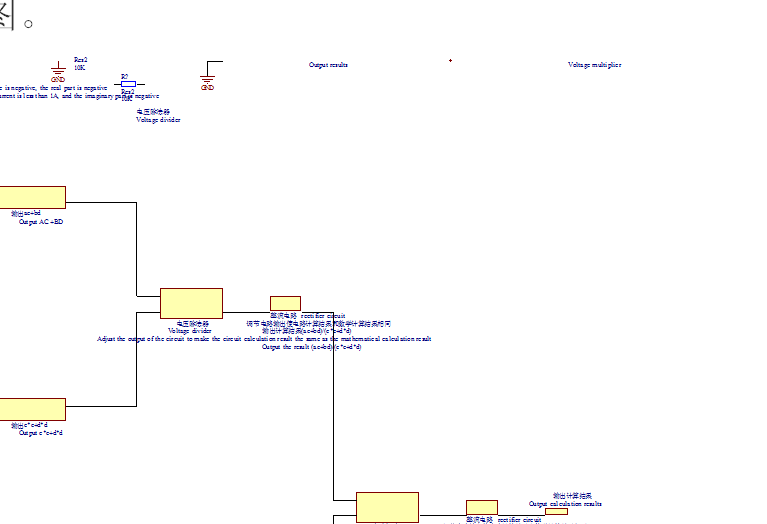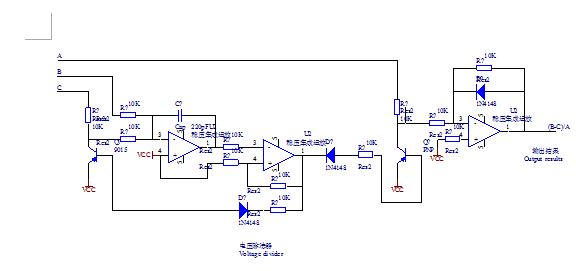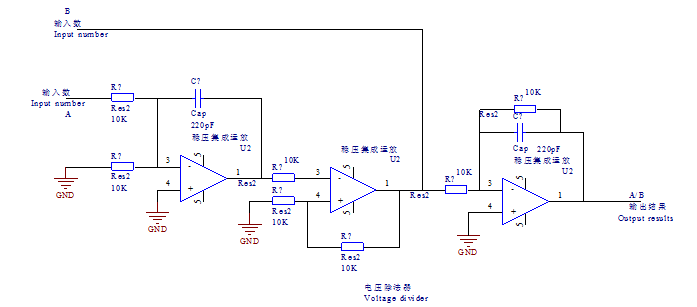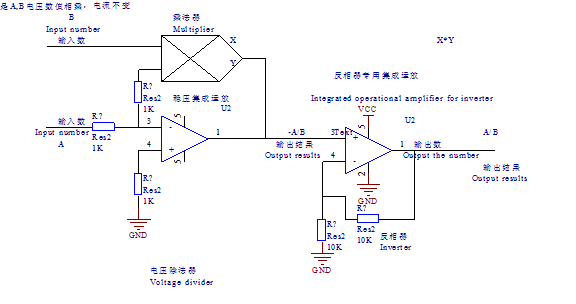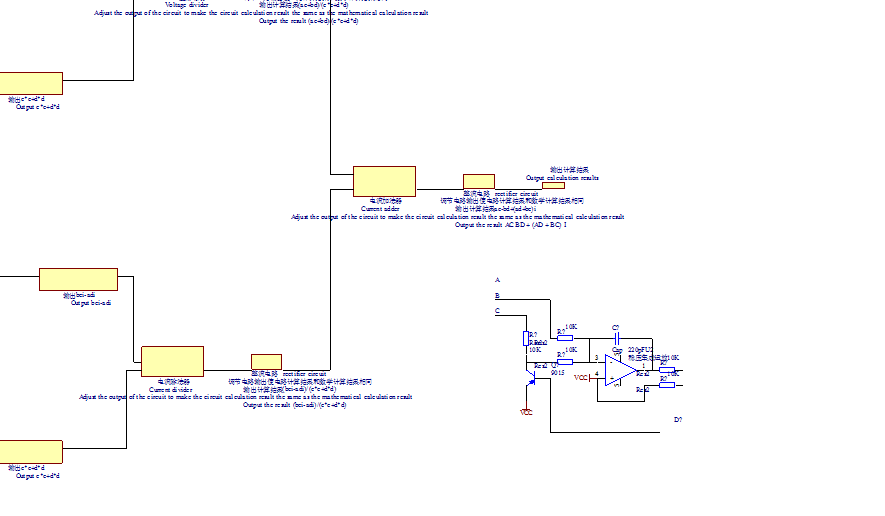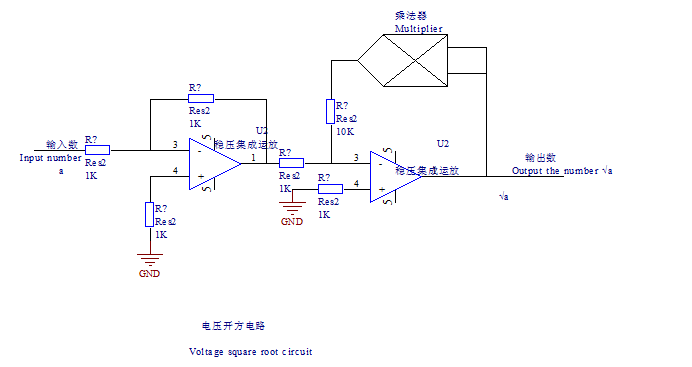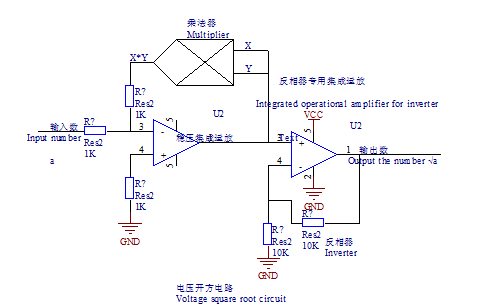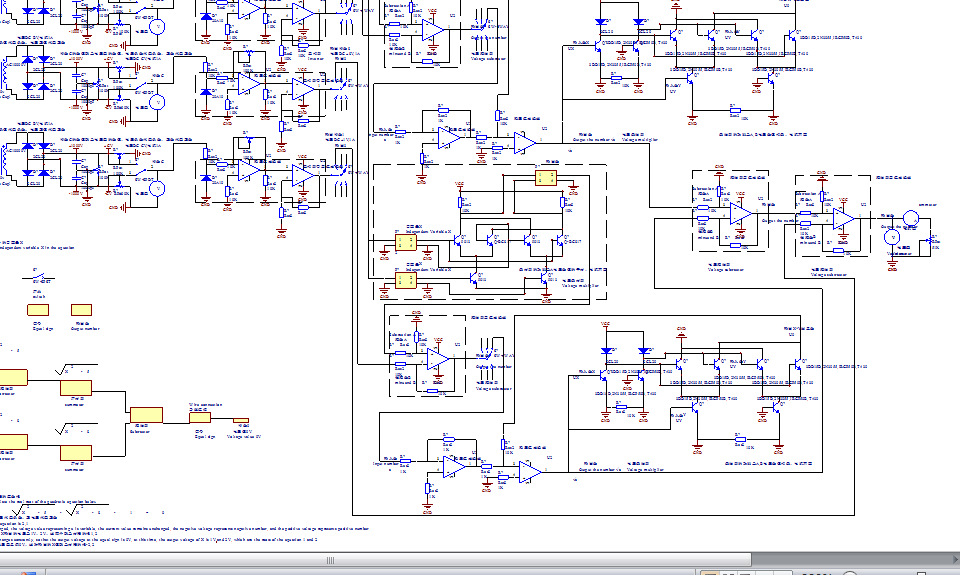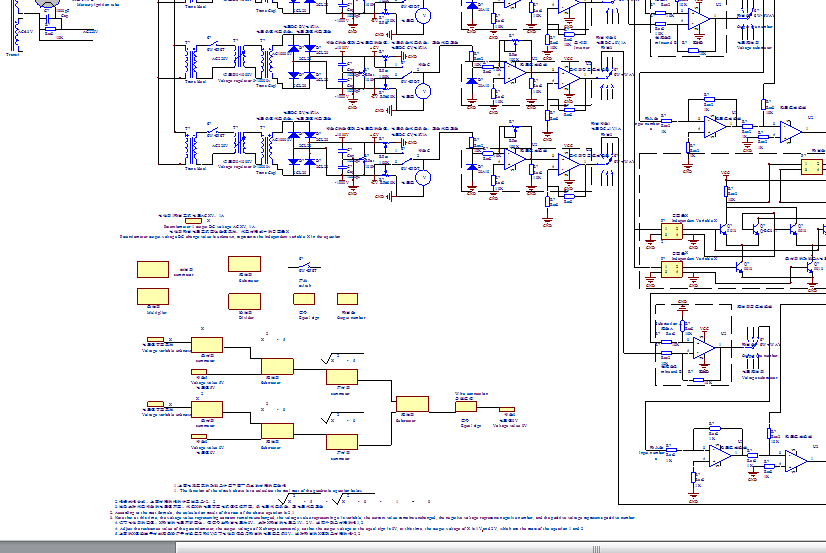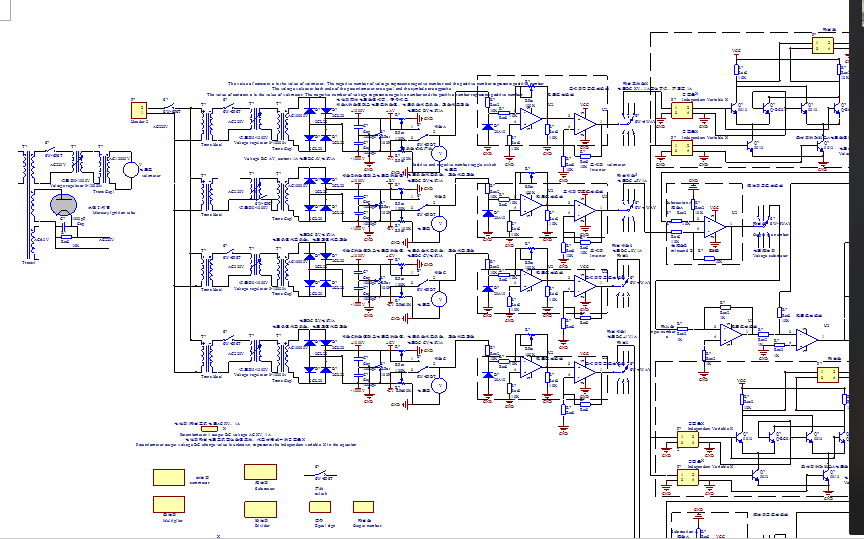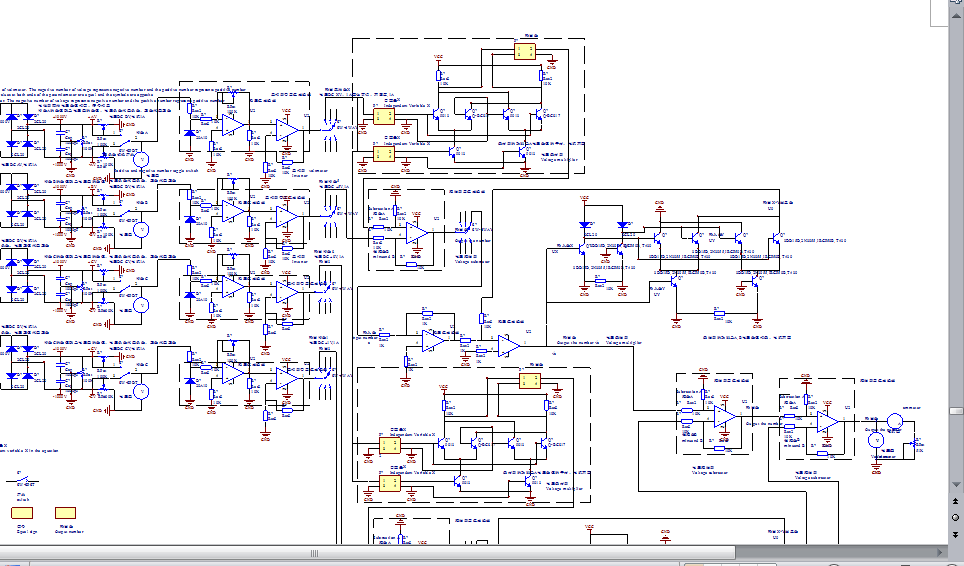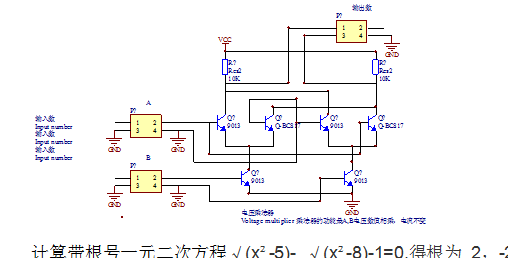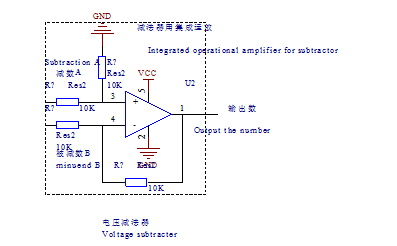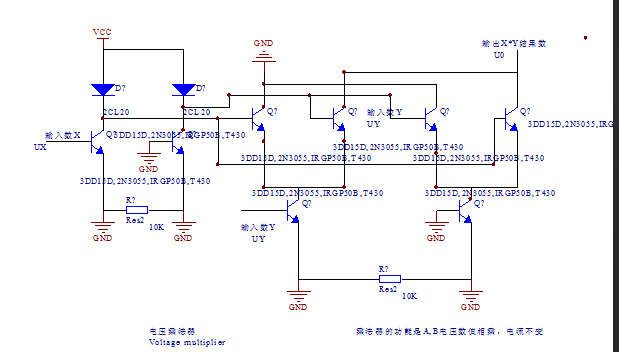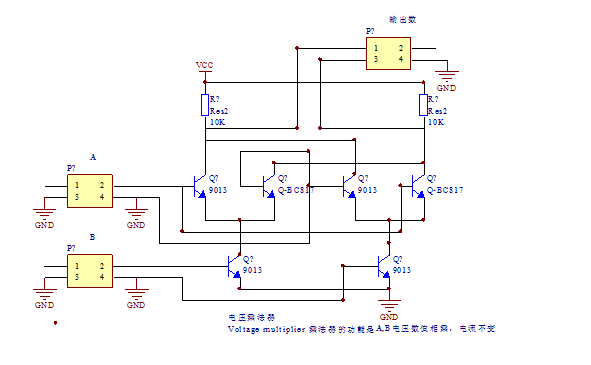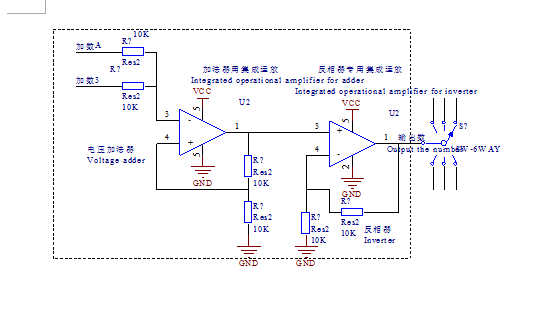展开全文• 满意答案JJ宋娇2017.01.09采纳率：54%等级：8已帮助：813人#include "stdio.h"#include "math.h"int main(void){int a,b,c;double d,e,f;scanf("%d %d %d",&...if (a==0)if (b==0){printf("非法方程，请...

满意答案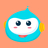JJ宋娇

2017.01.09采纳率：54%    等级：8

已帮助：813人

#include "stdio.h"

#include "math.h"

int main(void)

{int a,b,c;

double d,e,f;

scanf("%d %d %d",&a,&b,&c);

d=a, e=b, f=c;

if (a==0)

if (b==0)

{printf("非法方程，请重新输入a，b，c“);

scanf("%d %d %d",&a,&b,&c);d=a, e=b, f=c;}

else

if (b*b-4*a*c==0)

printf("x1=x2=%.6f\n",0-e/(2*d));

else

if (b*b-4*a*c>0)

printf("x1=%.6f\nx2=%.6f\n",(-e+sqrt(e*e-4*d*f))/(2*d),(-e-sqrt(e*e-4*d*f))/(2*d));

else

{

【5】;

【6】

printf("x1=%.6f+%.6fi\nx2=%.6f+%.6fi\n",-e/(2*d),sqrt(4*d*f-e*e)/(2*d),-e/(2*d),-sqrt(4*d*f-e*e)/(2*d));

}

5,6没法填

00分享举报

展开全文• 一元二次方程 ax²+bx+c=0 的包含3种情况(这里仅讨论a!=0的情况) #include<stdio.h> #include<math.h>void ax(int a,int b,int c); //δ>0void bx(int a,int b,int c); //δ=0void cx(int a,int b,...

一元二次方程 ax²+bx+c=0 的根包含3种情况
Δ>0
Δ=0
Δ<0
(这里仅讨论a!=0的情况)

#include<stdio.h>
#include<math.h>
void ax(int a,int b,int c); //Δ>0
void bx(int a,int b,int c); //Δ=0
void cx(int a,int b,int c); //Δ<0
int main()
{
int a,b,c;
scanf("%d%d%d",&a,&b,&c);
if(a<0) {
a=-a;
b=-b;
c=-c;
}
if(b*b-4*a*c>0)
ax(a,b,c);
if(b*b-4*a*c==0)
bx(a,b,c);
if(b*b-4*a*c<0)
cx(a,b,c);
return 0;
}
void ax(int a,int b,int c) {
double x0,x1,x2;
x0 = sqrt(b*b-4*a*c);
x1=(-b+x0)/(2.0*a);
x2=(-b-x0)/(2.0*a);
printf("x1=%.3f x2=%.3f\n",x1,x2);
}

void bx(int a,int b,int c) {
double x = -b/(2.0*a);
printf("x1=%.3f x2=%.3f\n",x,x);
}

void cx(int a,int b,int c) {

double m = -b/(2.0*a);
double n=sqrt(-(b*b-4*a*c))/(2.0*a);
printf("x1=%.3lf+%.3lfi x2=%.3lf-%.3lfi\n",m,n,m,n);
}


展开全文• 方程求解源代码，包括一元二次方程，一元三次方程，一元四次方程。使用求公式进行求解，解为复数解，二次方程总是有两个解，三次方程总是有三个解，四次方程总是有四个解。如果只需要实数解，可判断虚部是否为0。
• 羽墨志记录一切开心与不开心目录⊙一元二次方程⊙判别式⊙求公式⊙代码实现⊙运行结果前两天在《一元二次方程实数求解》一文中给出了一元二次方程在实数范围内的求解方法，换言之，方程复数范围内的求解方法...
• 求解一元二次方程，包含复数解 #include<stdio.h> #include<math.h> int main(){ float a,b,c,disc,com,fol; scanf("%f,%f,%f",&a,&b,&c); if(a==0) printf("不是二元一次方程!"); ...
• 实数解与复数解均可 #include<iostream> using namespace std; void Calculate(double a, double b, double c) { double m = b * b - 4 * a * c; if (m == 0) { cout << "该方程只有一个解"<...
• C++计算并输出一元二次方程 二次方程 ax2+bx+c = 0 (其中a≠0），a 是二次项系数，bx 叫作一次项，b是一次项系数；c叫作常数项。 x 的值为： 的判别式 示例 #include <iostream> #include <cmath&...
• 例子说明对于如下的一元二次方程：设计C语言程序，输入一元二次方程的三个系数a、b、c，求解出该方程的两个，并且允许用户在程序中多次输入不同的系数，以求解不同的一元二次方程的解。编程思路分析对于该方程，令...
• 一元二次方程ax2+bx+c=0，输入a,b,c三个系数，求解方程，结果有三种情况：两个实数、一个实数、两个复数根。/*** Equation.java*/package cn.edu.uibe.oop;/*** 计算一元二次方程* @author TongQiang**/...
• 本文使用Python实现一元二次方程公式，主要演示运算符和几个内置函数的用法，封面图片与本文内容无关。def root(a, b, c, highmiddle=True): #首...python 人工智能 机器学习
• 求解一元二次方程的思路 求解一元二次方程 ax2+bx+c=0ax^2+bx+c=0ax2+bx+c=0 的思路，将该方程转化为 (x−x′)2=K(x-x')^2=K(x−x′)2=K，得到 x=±K+x′x = \pm\sqrt{K}+x'x=±K​+x′ 所以有如下转化过程： ax2+bx...
• 因为Matlab能进行复数运算，所以可以直接求解一元二次方程。 叮~~ function [x]=equation(a,b,c) d=b*b-4*a*c; x=[(-b+sqrt(d))/(2*a),(-b-sqrt(d))/(2*a)]; 在命令行窗口输入并得求解结果 >> [x]=...matlab
• print('计算一元二次方程，请依次输入各个系数：') a=float(input('请输入a的值：')) b=float(input('请输入b的值：')) c=float(input('请输入c的值：')) d=float(b**2-4*a*c) #P(process):处理数据 if a!=0: ...
• = 0: delta = b**2-4*a*c if delta  print("无根") elif delta == 0: s = -b/(2*a) print("唯一的x=",s) else: root = math.sqrt(delta) x1 = (-b+root)/(2*a) x2 = (-b-root)/(2*a) print("x1=",x1,"\t","x2=",...python
• #include #include//sqrt函数需要使用该头文件 using namespace std;//必须得加上 int main() { float a,b,c,d; cout<<"a="<>a;... if(a==0) cout不是一元二次方程组"; else if(d>0) { cout方程有两个实"
• 那个时候老师就告诉我们，一元二次方程一般有两个，一元n次方程则一般有n个。后来在课外书上了解到，这个结论最早是由数学王子高斯严格证明出来的。当时我就有一个疑惑，明明有些一元二次方程因为判别式小于0是...
•python
• if(result) { printf("方程复数!\n"); } else { float x1=(-b+sqrt(result))/(2*a); printf("The first answer is %.2f.\n",x1); float x2=(-b-sqrt(result))/(2*a); printf("The second answer is %.2f.\n",...
• 一元二次方程ax2+bx+c=0，输入a,b,c三个系数，求解方程，结果有三种情况：两个实数、一个实数、两个复数根。/*** Equation.java*/package cn.edu.uibe.oop;/*** 计算一元二次方程* @author TongQiang**/...
• 共回答了15个问题采纳率：80%import java.util.*;class First{public static void main(String[] args)...System.out.println("请输入一元二次方程的三个系数a,b,c的值");System.out.println("请输入系数a的值");dou...
• ## 一元四次方程的求解

万次阅读 多人点赞 2019-11-09 10:59:20
一元次方程求解0.引言1.方法一2.方法3.结论 0.引言 在学习过程中需要求解一个一元次方程。 由于带有未知参数，本想使用MATLAB求解出它的解析解然后写入C++进行计算。后来发现求解出来的解太长。 要是直接放...一元四次方程求解
• 用c++编写求解一元二次方程的程序。包含了复数根求解
• 前言： 本人因喜欢python和c语言等程序设计语言，希望能够在这里和大家共同学习共同进步。...1.求一元二次方程，要求随机输入a,b,c的值，计算并输出方程 可以先自己看要求写出程序，然后看提示及答案，更...python
• 计算机语言运用--数值计算9-方程的计算机处理92-4_Fortran数值计算方程的计算机处理这里说的方程，是以实数域为计算范围。这里说的方程：是含有未知数的等式。可以是a+5=8，或X+A=B，或ax2+bx+c=0，或ax+by=c与dx-ey...
• a=0,不是一元二次方程； b^2-4ac=0,有两相等的实；x1=x2=-2a/b;b^2-4ac>0,有两不等的实；p=-b/2a,q=sqrt(b^2-4ac)/2a;x1=p+q,x2=p-q;b^2-4ac<0,有两个共轭复根；p=-b/2a,q=sqrt(4ac-b^2)/2a;x1=p+qi,x2=p-...
• 题目： 从键盘输入三个实数，输出实数复数根 代码： #include <stdio.h> #include <...void root(double a,double b,double c);... printf("请输入一元二次方程x-y-z的值："); scanf("%lf,%......